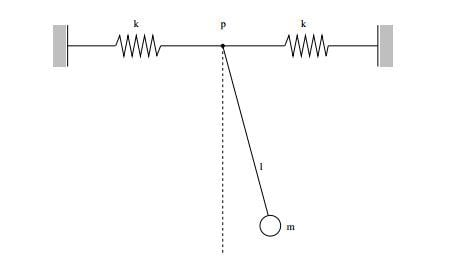# Mathemathical pendulum with springs

## Homework Statement

A body with mass ##m## is hanged on a line with length ##l## and attached to springs in point ##p##. Point ##p## can move only horizontally. In equilibrium position, non of the springs is deformed. Now let's give that body just a little push out of equilibrium position. Calculate the frequency of oscillation.## The Attempt at a Solution

Let's put the origin of our coordinate system in point ##p## with ##\hat{i}## axis to right along the right spring and ##\hat{j}## axis pointed up.

Now the coordinate is fixed, while point ##p## can move along axis ##\hat{i}##. Let ##\vec{r} ## be vector from the origin to mass ##m## and lets say that ##\varphi ## is the angle between the line ##l## and vertical line.

Than ##\vec{r}=(lsin\varphi + x,lcos\varphi )## where ##x## is the expansion/shrinkage of springs or in other words: movement of point ##p##.

Than ##\dot{\vec{r}}=(\dot{\varphi }lcos\varphi+\dot{x},-l\dot{\varphi }sin\varphi)## and ##\dot{\vec{r}}^2=(\dot{\varphi }l)^2+2\dot{\varphi }l\dot{x}cos\varphi+\dot{x}^2##.

Now ##L=T-V=\frac{1}{2}m\dot{\vec{r}}^2-(-mglcos\varphi +kx^2)=(\dot{\varphi }l)^2+2\dot{\varphi }l\dot{x}cos\varphi+\dot{x}^2+mglcos\varphi -kx^2##

If that is ok, than

##\frac{\partial L}{\partial \varphi }-\frac{\mathrm{d} }{\mathrm{d} t}\frac{\partial L}{\partial \dot{\varphi }}=\ddot{\varphi }+\frac{g}{l}sin\varphi +\frac{\ddot{x}}{l}cos\varphi =0## and

##\frac{\partial L}{\partial x}-\frac{\mathrm{d} }{\mathrm{d} t}\frac{\partial L}{\partial \dot{x }}=\ddot{x}+\frac{2k}{m}x+\ddot{\varphi }lcos\varphi -\dot{\varphi }^2lsin\varphi =0##

Hopefully so far everything is ok. Now I am lost here. I am somehow supposed to solve this system of differential equations yet I have no idea how. I tried applying Taylor expansion for small ##\varphi ## but that didn't really help much...

vanhees71
Gold Member
Taylor expanding for small $\varphi$ and $\dot{\varphi}$ helps enormously. Take the expansion up to linear order and you get a set of two coupled linear equations of motion which you can solve with standard methods, finding the eigensystem of solutions.

Yup, I figured it out yesterday late into the night...

For small angles:

##\ddot{\varphi }+\frac{g}{l}\varphi +\frac{1}{l}\ddot{x}=0## and

##\ddot{x}+\frac{2k}{m}x +l\ddot{\varphi }=0##

Now lets say that ##x=x_0e^{i\omega t}## and ##\varphi =\varphi _0e^{i\omega t}##. This gives me

##\begin{bmatrix}
-\omega ^2+\frac{g}{l} & -\omega ^2\\
-\omega ^2& -\omega^2 +\frac{2k}{m}
\end{bmatrix}\begin{bmatrix}
\varphi _0\\
x_0
\end{bmatrix}=\begin{bmatrix}
0\\
0
\end{bmatrix}##

And finally ##\omega ^2=\frac{g/l}{1+\frac{mg}{2kl}}##

THANKS!

vanhees71
Hm, aren't there two resonance frequencies? You must have a solution with four free parameters, determined by the initial conditions $x(0)=x_0$, $\dot{x}_0=v_0$, $\varphi(0)=\varphi_0$, and $\dot{\varphi}(0)=\Omega_0$.